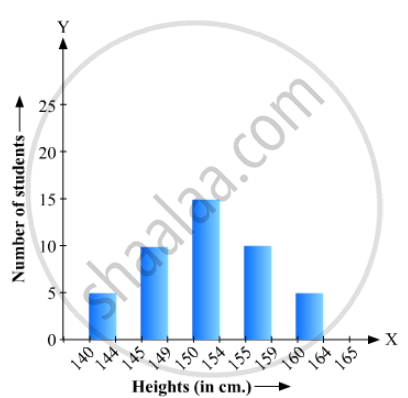# The Following Bar Graph (Fig. 23. 1 4) Represents the Heights (In Cm) of 50 Students of Class Xi of a Particular School. Study the Graph and Answer the Following Questions: - Mathematics

The following bar graph (Fig. 23. 1 4) represents the heights (in cm) of 50 students of Class XI of a particular school. Study the graph and answer the following questions:(i) What percentage of the total number of students have their heights more than 149cm?
(ii) How many students in the class are in the range of maximum height of the class?
(iii) The school wants to provide a particular type of tonic to each student below the height
of 150 cm to improve his height. If the cost of the tonic for each student comes out to be Rs. 55, how much amount of money is required?
(iv) How many students are in the range of shortest height of the class?
(v) State whether true or false:
a. There are 9 students in the class whose heights are in the range of 155 - 159 cm.
b. Maximum height (in cm) of a student in the class is 17.
c. There are 29 students in the class whose heights are in the range of 145- 154 cm.
d. Minimum height (in cm) of a student is the class is in the range of 140 – 144 cms.
e. The number of students in the class having their heights less than 150 cm is 12.
f. There are 14 students each of whom has height more than 154. cm.

#### Solution

(1) The total number of students is 50. The number of students having heights more than 149 cm is
Desired percentage is:

=(17+9+5)/50 xx 100

= 62%

(2) The maximum range of height is 164-165 cm. The number of students belonging to this group is 5.

(3) The number of students whose heights are less than 150 cm is 7 + 12 = 19. Hence, the total cost is 19 × 55= Rs 1045/-

(4) The minimum range of height is 140-144 cm. The number of students belonging to this group is 7.

(5) (a) The number of students whose heights are in the range 155-159 cm is 9. Hence, the statement is true.

(b) The maximum possible height (in cm) of a student in the class can be 164 cm. Hence, the statement is false.

(c) The number of students whose heights are in the range 145-154 cm is 12 +17 = 29 . Hence, the statement is true.

(d) The minimum range of heights of students in the class is 140-144 cm. Hence, the statement is true.

(e) The number of students having heights less than 150 cm is 7 +12+ 19 . Hence, the statement is false.

(f) The number of students having heights more than 154 cm is

9+5=14.  Hence, the statement is true.

Concept: Graphical Representation of Data
Is there an error in this question or solution?

#### APPEARS IN

RD Sharma Mathematics for Class 9
Chapter 23 Graphical Representation of Statistical Data
Exercise 23.1 | Q 10 | Page 12

Share